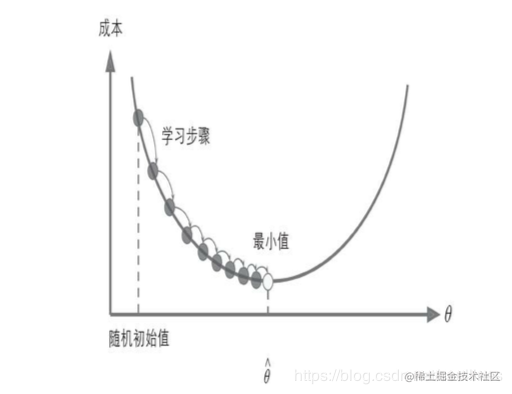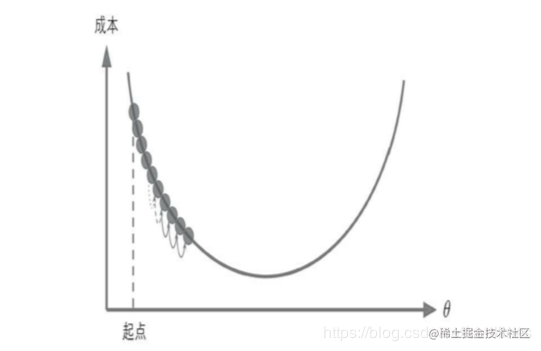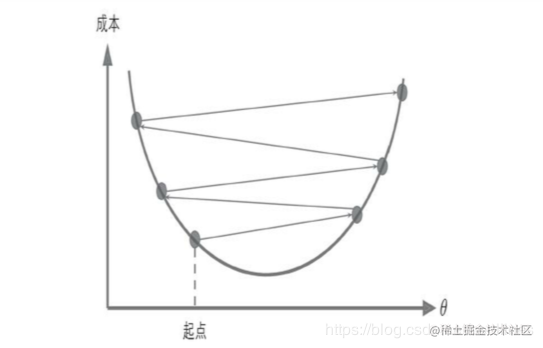# 从标准方程,梯度下降窥探机器学习训练原理

• 标准方程
• 梯度下降
进而来看基于以上两者如何实现模型的训练,基本思想一致,不同的模型大家可以触类旁通

1.标准方程

2.梯度下降

3.机器学习模型训练过程

#### 1.标准方程

• 1.线性回归的预测模型
y =θ ∗ X y=θ∗X y = \theta * X y = θ∗ X
• 2.那么线性回归的成本函数就是MSE:
M SE ( X ,h θ )= 1/m∑ m i =0 (θ T ∗X i−y i) 2 MSE(X,hθ)=1/m∑i=0m(θT∗Xi−yi)2 MSE(X,h_\theta) = 1/m \sum_{i=0}^{m}(\theta^T * Xi - yi)^2 M SE (X, h θ ​ )= 1/m i=0 ∑ m ​ (θ T ∗ X i− y i ) 2
• 3.接着我们对MSE求导,并令其等于0,这样得到一个权重theta为因变量的闭式解:
θ =( X T ∗X ) − 1 ∗X T ∗y θ=(XT∗X)−1∗XT∗y \theta = (X^T * X)^{-1} * X^T * y θ = ( X T ∗ X) −1 ∗ X T ∗ y
• 4.得到标准方程,模型便可以开始预测了:
y (pr ed )=θ ∗X y(pred)=θ∗X y(pred) = \theta * X y ( pred) = θ ∗ X

#### 2.梯度下降

θ (n e xt) =θ− η∂ ms e ∂ θ θ(next)=θ−η∂mse∂θ \theta(next) = \theta - \eta \frac{\partial mse}{\partial \theta} θ( next) = θ − η ∂θ ∂mse ​
θ :权 重向 量η :学 习率 ∂ ms e ∂ θ :梯 度 θ:权重向量η:学习率∂mse∂θ:梯度 \theta:权重向量 \quad \eta:学习率 \quad \frac{\partial mse}{\partial \theta}:梯度 θ : 权重向量 η: 学习率 ∂θ ∂m se ​ : 梯 度

• 梯度下降:通过上面的公式不断更新权重,不断的训练模型,当成本函数达到理想值的时候,停止更新权重,即停止训练模型##### 2.1:梯度向量的计算:

∂ ms e ∂ θ =2 /m∗ ∑ m i =1 (θ ∗X ( i) −y ( i) )x ( i) j ∂mse∂θ=2/m∗∑i=1m(θ∗X(i)−y(i))xj(i) \frac{\partial mse}{\partial \theta} = 2/m * \sum_{i=1}^m(\theta * X^{(i)} - y^{(i)})x_j^{(i)} ∂ θ ∂mse ​ = 2/m ∗ i=1 ∑ m ​ (θ∗ X (i) − y (i) )x j ( i) ​
∂ ms e ∂ θ =2 / m∗ X T ∗( X ∗ θ−y ) ∂mse∂θ=2/m∗XT∗(X∗θ−y) \frac{\partial mse}{\partial \theta} = 2/m * X^T * (X*\theta - y) ∂θ ∂ m se ​ =2 /m∗ X T ∗ (X ∗ θ − y )

##### 2.2:学习率1. 批量梯度下降:每次使用全部的数据进行梯度计算
2. 随机梯度下降:每次使用随机的一条数据进行梯度计算
3. 小批量梯度下降:每次从数据中随机取出一部分数据进行计算

#### 3.机器学习模型训练过程

m, n = housing.data.shape
n_epochs = 1000
learning_rate = 0.01
pipeline = Pipeline([
('std_scaler', StandardScaler())
])
scaled_housing_data_plus_bias = pipeline.fit_transform(housing_data_plus_bias)
serialize_data(scaled_housing_data_plus_bias,'scaled_housing_data_plus_bias')
X = tf.constant(scaled_housing_data_plus_bias, dtype=tf.float32, name='X')
y = tf.constant(housing.target.reshape(-1, 1), dtype=tf.float32, name='y')
# 获取初始的theta
theta = tf.Variable(tf.random_uniform([n + 1, 1], -1.0, 1.0), name='theta')
y_pred = tf.matmul(X, theta, name='predictions')
error = y_pred - y
mse = tf.reduce_mean(tf.square(error), name='mse')
gradients = 2 / m * tf.matmul(tf.transpose(X), error)
training_op = tf.assign(theta, theta - learning_rate * gradients)
# 开启会话
init = tf.global_variables_initializer()
with tf.Session() as sess:
sess.run(init)
for epoch in range(n_epochs):
if epoch % 100 == 0:
print('Epoch', epoch, 'MSE = ', mse.eval())
sess.run(training_op)
# training_op.eval()
best_theta = theta.eval()

1. 初始 化权 重向 量θ ,这 里的 θ初 始化 需要 注意 ,θ的 sha pe须 为( f eat u re s,) 1.初始化权重向量θ,这里的θ初始化需要注意,θ的shape须为(features,) 1.初始化权重向量\theta,这里的\theta初始化需要注意,\theta的shape须为(features,) 1 .初 始化权 重向量 θ ,这里 的 θ初始 化需要 注 意,θ 的shap e 须为( fea t ure s, )
2. 接 着 利 用 θ∗ X 求 得 当 前 的 预 测 值 ,进 而 求 的 M S E , 然 后 利 用 下 面 公 式 : 2.接着利用θ∗X求得当前的预测值,进而求的MSE,然后利用下面公式: 2.接着利用\theta*X求得当前的预测值,进而求的MSE,然后利用下面公式: 2. 接着 利用θ ∗ X求得 当 前的 预测值 , 进而求 的M S E , 然后 利用 下面 公式 : ∂ ms e ∂ θ = 2 / m ∗ X T ∗ ( X ∗ θ − y ) ∂mse∂θ=2/m∗XT∗(X∗θ−y) \frac{\partial mse}{\partial \theta} = 2/m * X^T * (X*\theta - y) ∂ θ ∂ m s e ​ = 2 / m ∗ X T ∗ ( X ∗ θ − y ) θ ( n e x t ) = θ − η ∂ m s e ∂ θ θ ( n e x t ) = θ − η ∂ m s e ∂ θ \theta(next) = \theta - \eta \frac{\partial mse}{\partial \theta} θ ( n e x t ) = θ − η ∂ θ ∂ m s e ​

eg:每次从数据中取出50条数据进行训练,这样效率大大提高